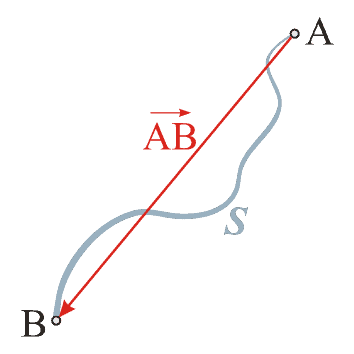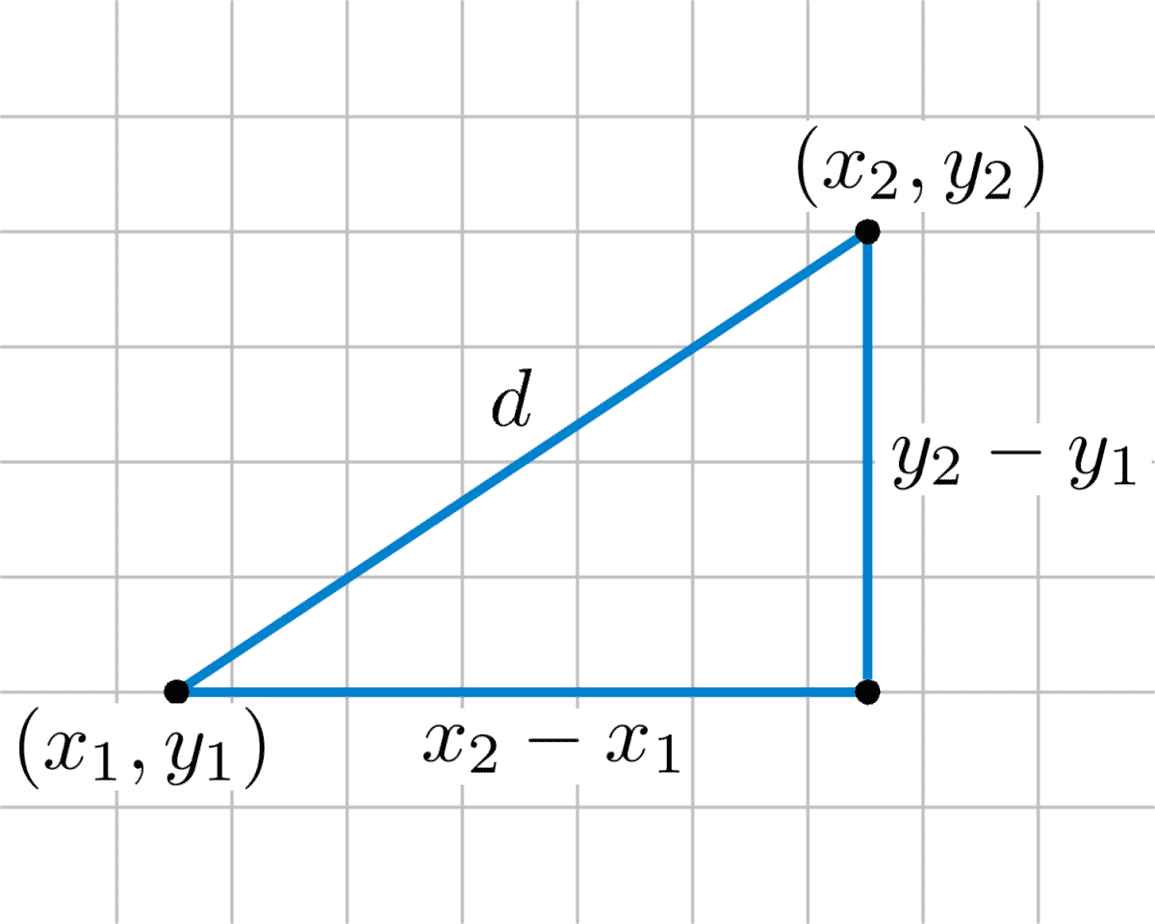# Can Distance Be Negative? (5 Common Distance Questions Answered)

Distance is used in math and physics to describe how far an object has traveled.  However, there are still some questions about distance that need to be answered – for example, its sign.

So, can distance be negative?  Distance cannot be negative, since it is a scalar quantity.  It measures the total distance traveled over a time period, without regard to direction or change in direction.  If you walk once around a circular track that is 1 mile long, the distance is 1 mile, but the displacement is 0 miles.

Of course, distance and displacement may be the same in some cases (such as when the direction of an object does not change).

Let’s get started.

## Can Distance Be Negative?

Distance cannot be negative.  By definition, distance is either

• A measure of how far an object has traveled in a given time period, or
• How far apart two objects are at a given point in timeDistance (blue line) tells us how far an object has traveled in total. Displacement (red line) tells us how far from the starting point an object is.

By either definition, distance cannot be negative, since:

• By the first definition, an object cannot travel a negative total distance in a given time period.  At the very least, it stays still and the distance traveled is zero.
• By the second definition, two objects cannot be a negative distance apart.  If they are in the same place, the distance is zero; otherwise, the distance is positive.

Distance can be measured in 1, 2, 3, or more dimensions.

## What Is The Distance Formula?

There are a few different formulas to find the distance between two points (or objects):

• 1-dimensional distance: D = |x2 – x1|  [this is the distance between two points x1 and x2 on a number line].
• 2-dimensional distance: D = √((x2 – x1)2 + (y2 – y1)2)  [this is the distance between two points (x1, y1) and (x2, y2) in a coordinate plane]. This formula comes from the Pythagorean Theorem.
• 3-dimensional distance: D = √((x2 – x1)2 + (y2 – y1)2 + (z2 – z1)2) [this is the distance between two points (x1, y1, z1) and (x2, y2, z2) in a 3D space].The distance formula in two dimensions assumes a right triangle and comes from the Pythagorean Theorem.

Example 1: Calculating Distance In 1 Dimension

Given the points x = 3 and x = 8 on a number line, the distance between them is:

• D = |x2 – x1|
• D = |8 – 3|
• D = |5|
• D = 5

Example 2: Calculating Distance In 2 Dimensions

Given the points (2, 4) and (7, 16) on a number line, the distance between them is:

• D = √((x2 – x1)2 + (y2 – y1)2)
• D = √((7 – 2)2 + (16 – 4)2)
• D = √((5)2 + (12)2)
• D = √(25 + 144)
• D = √(169)
• D = 13

Example 3: Calculating Distance In 3 Dimensions

Given the points (1, 2, 3) and (4, 6, 15) in a 3D space, the distance between them is:

• D = √((x2 – x1)2 + (y2 – y1)2 + (z2 – z1)2)
• D = √((4 – 1)2 + (6 – 2)2 + (15 – 3)2
• D = √((3)2 + (4)2 + (12)2)
• D = √(9 + 16 + 144)
• D = √(169)
• D = 13

Remember: since distance is defined as either an absolute value or a principal square root, it is always nonnegative (either positive or zero).

Note: there is also the formula D = RT, where D = distance, R = Rate (or speed), and T = Time.  However, this formula only applies when the rate R is constant (that is, when speed does not change).

## Is Distance Vector Or Scalar?

Distance is a scalar quantity.  This means that it does not have a sign – it is always positive or zero, and it is never negative.

Distance is simply a number that tells us either:

• How far an object has traveled (the distance between two points: the starting point and the ending point)
• How far apart two objects are (the distance between two points)

### Does Distance Have Direction?

Distance does not have direction.  Distance only tells us how far an object has traveled, not which direction (or directions) it traveled in.

However, there is another concept that takes direction into account.  Displacement has direction as well as magnitude, and the direction can be negative.

However, displacement “cancels out” positive and negative travel.  So, if you were to walk 1 mile north and then 1 mile south, you have:

• A distance of 2 miles (1 mile north + 1 mile south = 2 miles walked).
• A displacement of 0 miles (1 mile north and then 1 mile south means we end up at the starting point, which is 0 miles away from where we began walking).

• Distance = Work Done (how far you have walked)
• Displacement = Progress Made (how far from the starting point you have gotten)

For example, let’s say you are lost in the woods and walk around in a 3-mile circle for an hour.  Then:

• You have a distance of 3 miles (you did the work of walking 3 miles)
• You have a displacement of 0 miles (you did not make any progress in getting out of the woods – you are still lost, and back to the starting point – in fact, you are a bit more tired now, and probably worse off!)

### Can Distance & Displacement Be The Same?

We cannot really compare distance and displacement, since one is a scalar and the other is a vector. However, we can compare distance with the magnitude of displacement.

In that case, the distance and the magnitude of displacement can be the same in one specific case: when the object does not change direction.

#### Example 1: When Distance & Magnitude Of Displacement Are The Same

For example, if you walk 2 miles north without changing direction, you have:

• A distance of 2 miles (2 miles north means you have walked 2 miles total).
• A displacement of 2 miles (2 miles north means we end 2 miles north of the starting point, which is 2 miles away from where we began walking).

#### Example 2: When Distance & Magnitude Of Displacement Are Not The Same

As another example, let’s say you walk 3 miles north and then 4 miles west from your starting point.  In that case, you have:

• A distance of 7 miles (3 miles north + 4 miles west = 7 miles walked in total).
• A displacement of 5 miles (using the Pythagorean Theorem, we can find the distance D between the starting point and ending point: 32 + 42 = D2, or 9 + 16 = D2, or 25 = D2, which gives us a displacement of 5 miles).

Due to the change in direction, the distance is greater than the displacement.

### Can Distance Be Greater Than Displacement?

Distance can be greater than the magnitude of displacement, as we saw in the last example.  In fact, distance will be greater than the magnitude of displacement whenever an object changes direction during its movement.

## Conclusion

Now you know that distance cannot be negative, and you also know how distance is related to displacement.